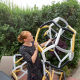# #MathArtChallenge Day 69: Another way to construct a rectangleA circle with a diameter. Multiple line segments connect from the end points of the diameter to another place on the circle.

The Challenge: Construct a rectangle using a compass.

Materials needed: Compass, straight edge, writing utensil
Math concepts you could explore with this challenge: angles, circles, geometric construction, geometry, lines, polygons, slope, symmetry, vertices/intersections

This also lends itself to the lovely drawing at the top of this post.

Depending on how you use this activity, you may engage with different mathematical standards. I’ve listed possible connected math content above. Here are a few suggestions for how you might integrate the 8 mathematical practices. Feel free to add your own suggestions in the comments!

3.) Construct viable arguments and critique the reasoning of others. Prove that any inscribed angle with endpoints that match the endpoints of a diameter creates a 90 degree angle.## Author: Ms. P

Math Teacher in Minneapolis, MN.

Posted on